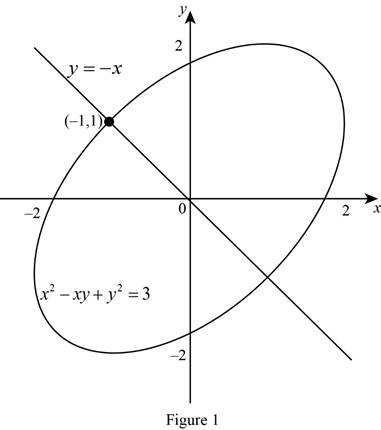# The point such that the normal line intersect the ellipse at second time and to sketch the equation of the ellipse and the normal line.### Single Variable Calculus: Concepts...

4th Edition
James Stewart
Publisher: Cengage Learning
ISBN: 9781337687805### Single Variable Calculus: Concepts...

4th Edition
James Stewart
Publisher: Cengage Learning
ISBN: 9781337687805

#### Solutions

Chapter 3.5, Problem 50E
To determine

## To find: The point such that the normal line intersect the ellipse at second time and to sketch the equation of the ellipse and the normal line.

Expert Solution

The normal line is intersect to the ellipse second time at the point (1,1).

### Explanation of Solution

Given:

The equation of the ellipse is x2xy+y2=3 and the point is (1,1).

Derivative rules:

Product rule: ddx(fg)=fddx(g)+gddx(f)

Chain rule: dydx=dydududx

Calculation:

Obtain the slope of the normal line to the ellipse.

Differentiate x2xy+y2=3 implicitly with respect to x,

ddx(x2xy+y2)=ddx(3)ddx(x2)ddx(xy)+ddx(y2)=ddx(3)2xddx(xy)+ddx(y2)=0

Apply the product rule and the chain rule,

2xddx(xy)+ddx(y2)=02x[xddx(y)+yddx(x)]+ddy(y2)dydx=02x[xdydx+y]+2ydydx=02xyxdydx+2ydydx=0

Combine the dydx on one side of the equation,

(2yx)dydx=y2xdydx=y2x2yx

Thus, the derivative of the equation is dydx=y2x2yx.

The slope of the tangent to the ellipse at (1,1) is computed as follows,

m=y2x2yx

Substitute (1,1) for (x,y),

m=12(1)2(1)(1)=1+22+1=1

Thus, the slope of the tangent to the ellipse at (1,1) is m = 1.

Note that, if the slope of the tangent line at (x1,y1) is m then the slope of the normal line at (x1,y1) is 1m.

Thus, the slope of the normal line to the ellipse at (1,1) is 1.

Obtain the equation of the normal at (1,1) and slope 1.

y1=1(x+1)y1=x1y=x

It is required that the normal line is intersect to the ellipse at second time.

Substitute y=x in x2xy+y2=3 to obtain the points.

x2x(x)+(x)2=3x2+x2+x2=33x2=3x=±1

Substitute x=±1 in y=x and obtain the values y=±1.

Thus, the points (1,1) and (1,1).

Therefore, the normal line is intersect to the ellipse at second time at (1,1).

Graph:

Use online graphing calculator to sketch the equation of the ellipse and the normal line as shown in Figure 1.From Figure 1, it is observed that the normal line y=x to the ellipse at the point (1,1) and it also intersect second time to the ellipse at the point (1,1).

### Have a homework question?

Subscribe to bartleby learn! Ask subject matter experts 30 homework questions each month. Plus, you’ll have access to millions of step-by-step textbook answers!